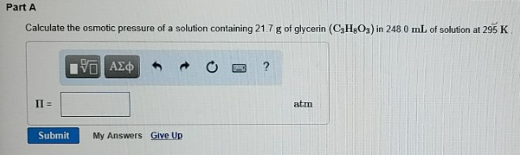# Problem: Calculate the osmotic pressure of a solution containing 21.7 g of glycerin (C3H8O3) in 248 0 ml, of solution at 295 K.

###### FREE Expert Solution

Where M is molarity (mol/L); i is van't Hoff factor; R is 8.314 (universal gas constant) and T is temperature

82% (232 ratings)###### Problem Details

Calculate the osmotic pressure of a solution containing 21.7 g of glycerin (C3H8O3) in 248 0 ml, of solution at 295 K.